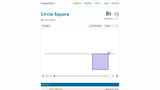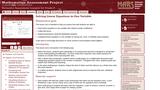WiMATHS

18 affiliated resources
View
Selected filters:Unrestricted Use
CC BY
Rating

This resource could be used an a precurser for a lesson on ...

This resource could be used an a precurser for a lesson on comparing areas of circles and squares. There is guess and check involved, as well as digging deeper to find the correct answer. Students could do part of this on their own device, using GeoGebra to help with graphing the equations. If students do not have access to devices, then the teacher can use it as a class discussion.

This includes questions that can be posed to students, as well as visuals to make the entire task make sense to students. Answer is included.

Subject:
Mathematics
Material Type:
Alternate Assessment
Interactive
Simulation
Provider:
Dan Meyer
04/18/2016Unrestricted Use
Public Domain
Rating

In this two-day lesson, students consider ways to estimate the number of ...

In this two-day lesson, students consider ways to estimate the number of spheres that will fit within a container. They also will try to pack as many as possible into differently shaped containers. The objective of this lesson is to have students use geometric solids so that they can solve basic packing problems that arise in the real world.

Subject:
Mathematics
Material Type:
Activity/Lab
Assessment Item
Formative Assessment
Interactive
Lesson Plan
Provider:
Indian Institute of Technology Gandhinagar
04/30/2016Unrestricted Use
CC BY
Rating

Students are given an arbitray unit circle and then asked the following ...

Students are given an arbitray unit circle and then asked the following questions:

Explain why sin(Ã¢Ë†â€™ÃŽÂ¸)=Ã¢Ë†â€™sinÃŽÂ¸ and cos(Ã¢Ë†â€™ÃŽÂ¸)=cosÃŽÂ¸. Do these equations hold for any angle ÃŽÂ¸? Explain.

Explain why sin(2Ãâ‚¬+ÃŽÂ¸)=sinÃŽÂ¸ and cos(2Ãâ‚¬+ÃŽÂ¸)=cosÃŽÂ¸. Do these equations hold for any angle ÃŽÂ¸? Explain.

This task can be used as a short formative assessment, and can be done individually, in pairs, or in small group.

Subject:
Mathematics
Material Type:
Assessment Item
Formative Assessment
Provider:
Illustrative Mathematics
04/18/2016Unrestricted Use
Public Domain
Rating

The source is an entire unit with formative assessments as well as ...

The source is an entire unit with formative assessments as well as the performance task.

Subject:
Mathematics
Material Type:
Alternate Assessment
Assessment Item
Formative Assessment
Full Course
Interim/Summative Assessment
Rubric/Scoring Guide
Unit of Study
Provider:
NYC Department of Education
04/18/2016Restricted Use
Rating

The resource is complete with worksheet, rubric, sample student work, and trouble ...

The resource is complete with worksheet, rubric, sample student work, and trouble shooting suggestions for the teacher.

Subject:
Mathematics
Material Type:
Assessment Item
Formative Assessment
Rubric/Scoring Guide
Provider:
Noyce Foundation
Provider Set:
Inside Mathematics
04/18/2016Unrestricted Use
CC BY
Rating

After playing a video clip of the Scarecrow from the Wizard of ...

After playing a video clip of the Scarecrow from the Wizard of Oz, just after he was 'given brains' and he mentions how the Pythagorean Theorem works have students look for precision in his statement.  This site helps you challenge students to be critical mathematicians and make the Scarecrow's statement more precise.  A link to the video clip is embedded on the page.

Subject:
Mathematics
Material Type:
Diagram/Illustration
Formative Assessment
Lesson Plan
Provider:
Glenrock Consulting, LLC
05/04/2016Restricted Use
Rating

This is a really nice task as it is open to everyone, ...

This is a really nice task as it is open to everyone, can be solved in different ways and can also extend to work in combinatorics Ã¢â‚¬â€œ a nice way of organizing counting. Ask students to work on this task in groups, and to display their results on posters. Often we name students' different approaches and strategies.

Subject:
Mathematics
Material Type:
Lesson Plan
Provider:
youcubed at Stanford University
04/18/2016Restricted Use
Rating

Students use data on space debris, analysis of rates, and regression in ...

Students use data on space debris, analysis of rates,  and regression in order to model situations.

Subject:
Mathematics
Material Type:
Alternate Assessment
Interim/Summative Assessment
Lesson Plan
Provider:
NCTM
04/18/2016Unrestricted Use
CC BY
Rating

This task presents a contextual situation in which algae is growing in ...

This task presents a contextual situation in which algae is growing in a lake at a rate of doubling every day.  The task asks students to evaluate claims about the extent to which the algae will grow and to create an equation that models the algae growth on the lake.

Subject:
Mathematics
Material Type:
Provider:
Illustrative Mathematics
04/18/2016Unrestricted Use
CC BY
Rating

This is a 3 act lesson by Dan Meyer. In this lesson ...

This is a 3 act lesson by Dan Meyer.  In this lesson students ask and answer, "How long does it take the sink to fill up?", after viewing a video of a leaky fauct.

Subject:
Mathematics
Material Type:
Diagram/Illustration
Lesson Plan
Simulation
Provider:
Dan Meyer
04/18/2016Restricted Use
Rating

This activity asks students to explore cubes with dimensions 1x1x1 up to ...

This activity asks students to explore cubes with dimensions 1x1x1 up to 4x4x4.  Each cube is painted on all six faces and students are asked to determine how many 1x1x1 cubes have zero painted faces, one painted face, two painted faces and three painted faces.  This activiy requires students to consider volume vs. surface area and also offers students the opportunity to predict and make genrealizations using patterns that are noticed.

Subject:
Mathematics
Material Type:
Activity/Lab
Diagram/Illustration
Formative Assessment
Interactive
Provider:
Youcubed.org
04/18/2016Unrestricted Use
CC BY
Rating

This is a high school geometry task that has students physically construct ...

This is a high school geometry task that has students physically construct the point equidistant from three non-collinear points and to identify why the construction works.  This construction motivates the notion of a triangle inscribed into a circle and why that particular construction might be useful.

This task is a procedures with connections task, of high cognitive demand.  The procedure is not specified for students but there is largely only one way of folding the paper to be able to identify the intersection point.  The high cognitive demand comes from students having to explain why the construction works and why only two creases are necessary.  This gets at both the meaning and motivation for the construction and the notion of efficiency in having a canonical construction for a circle that inscribes a triangle given three non-collinear points that can form a triangle.

This task could also be used as an assessment task after students learn the construction, although the explanations that may be given by students are more likely to focus on the construction procedures in this particular case.

This task addresses the Pivotal Understanding of equivalence, because it focuses on generating a geometric construction procedure that determines a point equidistant from three non-collinear points.  Equivalence is evident in at least two ways.  First, the distance from the target point to each of the source points is equal.  Second, the construction produces equivalent results (inscribed triangle within a circle given three points) each time.

Subject:
Mathematics
Material Type:
Assessment Item
Formative Assessment
Provider:
Illustrative Mathematics
12/21/2015Rating

The Posters problem explores an easily accessible real world issue of disecting ...

The Posters problem explores an easily accessible real world issue of disecting a single square piece of poster board into three rectangular pieces where the area of the pieces are related. Â The student has to determine what the relationships are based on the given information and determine the dimensions of each poster. Â Students can use a variety of strategies to approach this problem. Â A diagram is a good starting point and a persistent student could use a guess and check strategy. Â Knowledge of finding area is essential. Â This task reinforces writing and solving equations and systems. Â A suggested rubric is included for scoring the problem. Â

Subject:
Mathematics
Material Type:
Activity/Lab
Alternate Assessment
Assessment Item
Curriculum Map
Formative Assessment
Lesson
Lesson Plan
Rubric/Scoring Guide
Simulation
Provider:
Oregon Department of Education
01/01/2016Unrestricted Use
CC BY
Rating

Students will be working collaboratively with others to categorize equations into having ...

Students will be working collaboratively with others to categorize equations into having one, no, or infinite solutions. Students will then make generalizations about the characteristics of equations that have one, no, or infinite solutions.
This lesson unit is also intended to help you assess how well students are able to:
Ã¢â‚¬Â¢ Solve linear equations in one variable with rational number coefficients.Ã¢â‚¬Â¢ Collect like terms.Ã¢â‚¬Â¢ Expand expressions using the distributive property.
It also aims to encourage discussion on some common misconceptions about solving equations.

Subject:
Mathematics
Material Type:
Formative Assessment
Lesson Plan
Provider:
Mathematics Assessment Project
04/18/2016Unrestricted Use
Public Domain
Rating

The is a 3-Act lesson which shows a video of a man ...

The is a 3-Act lesson which shows a video of a man running Stairs and then running Super-Stairs.  Students generate questions like "How long will it take for him to run"  and "How many steps does he take".

This is appropriate for students studying sequences and series or as an enrichment problem for younger math students.

Subject:
Mathematics
Material Type:
Formative Assessment
Provider:
None
04/18/2016Restricted Use
Rating

There are three very different pieces of thinking needed to solve this ...

There are three very different pieces of thinking needed to solve this task.
For the first part, students need to combine or synthesize lots of learning about geometry, 3-dimensional shapes, and finding volume to derive a formula.
For the second part, students need to do a conversion from seconds to hours and minutes. To complete this part of the task, students need to understand the relationship between a decimal quantity and a numerical value when decimal is parts of 60 instead of parts of 100.
Students are being asked to synthesize previous knowledge to make sense of a less familiar application.
The final part is reasoning about a time graph by looking at a geometric shape and thinking about how a pool would fill.

Subject:
Mathematics
Material Type:
Alternate Assessment
Formative Assessment
Rubric/Scoring Guide
Provider:
Noyce Foundation
Provider Set:
Inside Mathematics
04/18/2016Unrestricted Use
CC BY
Rating

This is a rigrious lesson on finding the volume of cones and ...

This is a rigrious lesson on finding the volume of cones and cylinders.  A student should have a strong understanding of algebra to complete the tasks necessary in this lesson.

Subject:
Mathematics
Material Type:
Provider:
National Math and Science Initiative
04/18/2016Restricted Use
Rating

This task helps students build number sense as they practice calculating. The ...

This task helps students build number sense as they practice calculating. The task has more than one solution which is nice. At the end it asks students to write a clue that gives the task only one solution.

Subject:
Mathematics
Material Type: